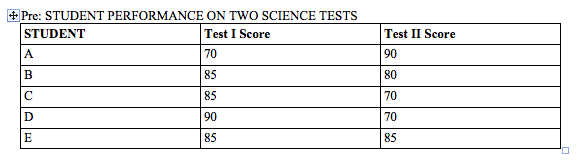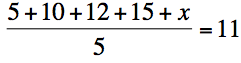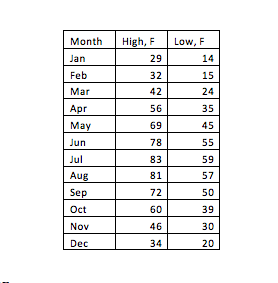Example Questions

← Previous 1 3

Example Question #75 : StatisticsThe chart above shows the scores on two tests for five students in a science class. If John's score was equal to the mode for Test I and equal to the median for Test II, then which of the five students represents John's scores?

E

C

B

D

A

B

Explanation:

The mode of a set of numbers is the number that appears most frequently in that set. The median of a set of numbers is the number that appears in the middle when they are arranged in numerical order. For Test I, the mode is 85. When arranged in numerical order, the scores for Test II are:

70, 70, 80, 85, 90.

That makes the median for Test II 80. The only student in the chart with an 85 on Test I and an 80 on Test II is student B.

Example Question #76 : Statistics

The average (arithmetic mean) of 1, 7, 9, and n is –1. What is the median of this set?

–2

7

1

4

–4

4

Explanation:

If the mean is –1, then 1 + 7 + 9 + n = –4. Solving for n gives us n = –21. In numeric order, we now have –21, 1, 7, 9. As there is an even number of numbers, we average the middle two. (1 + 7) / 2 = 4.

Example Question #77 : Statistics

The average of 5, 10, 12, 15, and x is 11. What is the median?

13

15

11

12

12

Explanation:

You must first find what x is in order to find the median.

To find x, set up the following equation:To solve the equation, first multiply both sides by 5:

5 + 10 + 12 + 15 + x = 55

Then, add up 5 + 10 + 12 + 15 to get 42:

42 + x = 55

x = 13

Now that you know what x is, you are ready to find the median. To find the median, order the numbers from lowest to highest. The median is the number in the middle.

5, 10, 12, 13, 15

Example Question #78 : StatisticsThe monthly averaged high-and low-temperatures in Michigan are shown in the given table. What are the median monthly averaged high- and low-temperatures respectively in Michigan?

high: 56F, low: 35F

high: 58F, low: 37F

high: 46F, low: 35F

high: 56F, low: 30F

high: 60F, low: 39F

high: 58F, low: 37F

Explanation:

Median is the middle number when the data are ordered from least to greatest. In this case, there are 12 numbers, so, the median is the average of the middle two numbers, which are the 6th and 7th numbers in an ascending order. For the averaged high-temperature, the median is (56+60)/2=58, and for the averaged low-temperature, the median is (35+39)/2=37

Example Question #79 : Statistics

Find the median of the following sequence:

3, 4, 5, 6, 7, 7, 10

5

6

10

7

6

Explanation:

The median is the middle number in a sequence when the numbers are put in order. Since this sequence is already in order from least to greatest, we just need to find the middle number. There are 7 terms so the middle term is (7+1)/2 or the 4th term. This is 6.

Example Question #80 : Statistics

Find the median of the data set:

25, 37, 13, 58, 52, 83, 21, 51

83

44

42.5

13

70

44

Explanation:

42.5 is the mean of the data.  13 is the minimum.  83 is the maximum.  70 is the range.

To find the median, list all numbers in order:

13, 21, 25, 37, 51, 52, 58, 83

and find the middle value.  In cases like this where there are two middle numbers (37 and 51), find the mean of these two numbers.

(37+51)/2 = 88/2 = 44

Example Question #1 : How To Find Median

Find the median in this set of numbers

2, 100, 52, 97, 1, 7, 22

97

22

1

7

52

22

Explanation:

in order to find the median, arrange the numbers in ascending order, and find the number in the middle of the list

Example Question #2 : How To Find Median

Find the median from the given set of numbers

1, 4, 8, 17, 8, 8, 15, 21, 32, 17

11.5

15

4

7

8

11.5

Explanation:

In order to find the median, arrange the numbers from smallest to biggest and find the number in the middle of the set.

In this case, both the 8 and 15 are in the middle of the set; so take the average of those 2 numbers (add both and divide by 2)

Example Question #3 : How To Find Median

The number is to be added to the list  {3, 4, 5, 6, 10, 12}. If is an integer, which of the following could be the median of the new list of seven numbers?

I) 5

II) 5.5

III) 6

I only

I and III only

I and II only

II and III only

I, II, and III

I and III only

Explanation:

Before is added to the list, the median is 5.5 (the average of 5 and 6). When n is added to the list, the number of elements becomes odd, so the median will be a value directly from the list, not the average of two values. All of the values in the old list are integers and n  is an integer, so the new median must be an integer; therefore, 5.5 cannot be the median of the new list.

Considering some possible values of n, we see that in cases where n is less than or equal to 5, the fourth element in the new list would be 5, making the new median 5. In cases where n  is greater than or equal to 6, the fourth element in the new list would be 6, making the new median 6. The possible values for the median of the new list are therefore 5 and 6, but not 5.5.

Example Question #4 : How To Find Median

M is a set consisting of a finite number of consecutive integers. If the average (arithmetic mean) of the numbers in set M is equal to one of the numbers in set M, which of the following must be true?

I. The number of numbers in set M is odd.

II. The average (arithmetic mean) of the numbers in set M equals the median.

III. Set M has a unique mode.

I only
I and II only
I and III only
I, II, and III
II only
Correct answer: I and II only
Explanation:

Statement I must be true because if M had an even number of consecutive integers, then its average (and median) would be the average of two consecutive integers, which is a decimal value rather than an integer, and therefore cannot be in set M, which contains only integers.

To check Statement II, consider some simple possible sets for M such as {0,1,2}. We see that in a set with an odd number of items, like M, the median is always the middle element. We also see that in a set with an odd number of consecutive integers (such as {0,1,2}), the average of the set will always be the exact middle element, too. Therefore, the average and the median must be equal, and Statement II must be true.

Finally, we can find a counterexample to statement III to show that it does not have to be true. If set M is  {0,1,2}, we see that there is no unique mode (in fact, the only time set M could have a unique mode is when it only has one element!)

← Previous 1 3